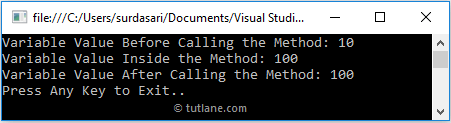# C# Pass By Reference (Ref) with Examples

In c#, passing a value type parameter to a method by reference means passing a reference of the variable to the method. So the changes made to the parameter inside the called method will affect the original data stored in the argument variable.

Using the `ref` keyword, we can pass parameters reference-type. It’s mandatory to initialize the variable value before passing it as an argument to the method in the c# programming language.

As discussed earlier, value-type variables will contain the value directly on its memory, and reference-type variables will contain a reference of its data.

## Declaration of C# Pass By Reference

Following is a simple example of passing parameters by reference in the c# programming language.

int x = 10; // Variable need to be initialized
Multiplication(ref x);

If you observe the above declaration, we declared and assigned a value to the variable x before passing it as an argument to the method by using reference (`ref`).

To use the `ref` parameter in the c# application, both the method definition and the calling method must explicitly use the `ref` keyword.

## C# Passing Parameters By Reference Example

Following is the example of passing a value type parameter to a method by reference in the c# programming language.

using System;

namespace Tutlane
{
class Program
{
static void Main(string[] args)
{
int x = 10;
Console.WriteLine("Variable Value Before Calling the Method: {0}", x);
Multiplication(ref x);
Console.WriteLine("Variable Value After Calling the Method: {0}", x);
Console.WriteLine("Press Enter Key to Exit..");
}
public static void Multiplication(ref int a)
{
a *= a;
Console.WriteLine("Variable Value Inside the Method: {0}", a);
}
}
}

If you observe the above example, we are passing the reference of variable x to variable a in the Multiplication method by using the ref keyword. In this case, the variable contains the reference of variable x, so the changes made to variable a will affect variable x.

## Output of C# Passing Parameters By Reference Example

When we execute the above c# program, we will get the result as shown below.If you observe the above result, the changes we did for the variable in the called method have also reflected the calling method.

This is how we can pass parameters to the method by reference using the `ref` keyword in c# programming language based on our requirements.